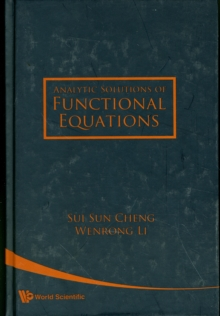Supporting your high street Find out how »
• My AccountAnalytic Solutions Of Functional Equations Hardback

Description

This book presents a self-contained and unified introduction to the properties of analytic functions.

Based on recent research results, it provides many examples of functional equations to show how analytic solutions can be found.Unlike in other books, analytic functions are treated here as those generated by sequences with positive radii of convergence.

By developing operational means for handling sequences, functional equations can then be transformed into recurrence relations or difference equations in a straightforward manner.

Their solutions can also be found either by qualitative means or by computation.

The subsequent formal power series function can then be asserted as a true solution once convergence is established by various convergence tests and majorization techniques.

Functional equations in this book may also be functional differential equations or iterative equations, which are different from the differential equations studied in standard textbooks since composition of known or unknown functions are involved.

Information

• Format: Hardback
• Pages: 296 pages
• Publisher: World Scientific Publishing Co Pte Ltd
• Publication Date:
• Category: Calculus & mathematical analysis
• ISBN: 9789812793348

£100.00

£86.95

on all orders

Pick up orders

from local bookshops